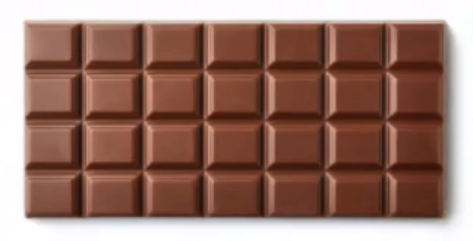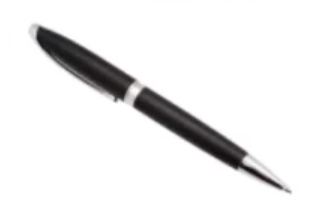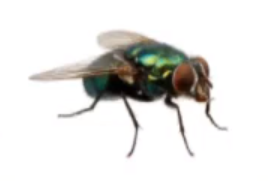What unit would be used to measure the length of the following?What unit would be used to measure the length of the following?What is likely to be the length of the following?What is likely to be the length of the following?What unit would you use to measure the length of the following?